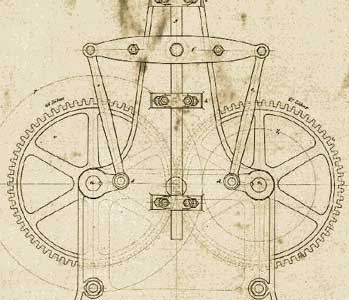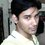# Six Questions To Test Kinematics.Apply yourself

1. Determine the absolute value of the velocity of a particle at time t=2s if the particle moves according to the law $\displaystyle r=\alpha { t }^{ 2 }\hat { i } +\beta (\sin { \pi t } )\hat { j }$, with $\displaystyle \alpha =2{ m }/{ { s }^{ 2 } }$ and $\displaystyle \beta =3m$

2. A particle moves according to the law $\displaystyle r=\alpha \sin { 5t } \hat { i } +\beta ({ cos }^{ 2 }5t)\hat { j }$ with $\displaystyle \alpha =2m$ and $\displaystyle \beta =3m$.Find the velocity vector, acceleration vector, and trajectory of the particle's motion.

3. The velocity of a particle varies according to the law $\displaystyle v=\alpha (2{ t }^{ 3 }-\beta )\hat { i } -\gamma (\sin { 2\pi t/3 } )\hat { j }$ with $\displaystyle \alpha =1{ m }/{ { s }^{ 4 } },\quad \beta =1{ s }^{ 3 },\quad \gamma =1{ m }/{ s }$. Find the law of motion if at the initial moment t=0 the particle was at origin.

4. The acceleration of a particle varies according to the law $\displaystyle a=\alpha { t }^{ 2 }\hat { i } -\beta \hat { j }$ with $\displaystyle \alpha =3{ m }/{ { s }^{ 4 } }$ and $\displaystyle \beta =3{ m }/{ { s }^{ 2 } }$. Find the distance from the origin to the point where the particle will be at time t=1s if at t=0 the particle is at origin and has zero velocity.

5. A train is moving rectilinearly with a velocity $\displaystyle \upsilon =180{ km }/{ hr }$ . Suddenly an obstacle appears on the tracks and the brakes are applied.Now the velocity of the train changes according to $\displaystyle \nu =\upsilon -\alpha { t }^{ 2 }$ with $\displaystyle \alpha =1{ m }/{ { s }^{ 2 } }$ . What is the breaking distance of the train?How much time must pass from the moment the brakes are applied before the train stops?

6. A rocket is launched from a land-based site vertically with an acceleration $\displaystyle a=\alpha { t }^{ 2 }$ , with $\displaystyle \alpha =1{ m }/{ { s }^{ 4 } }$ . At an altitude 100km above Earth's surface the rocket's boosters fail. How much time will pass (from the moment the boosters failed) before the rocket crashes? Air drag is ignored. The initial velocity is 0.

Don't forget to explain yourself in comments.

Have a nice day.

Want More?Note by Soumo Mukherjee
6 years, 9 months ago

This discussion board is a place to discuss our Daily Challenges and the math and science related to those challenges. Explanations are more than just a solution — they should explain the steps and thinking strategies that you used to obtain the solution. Comments should further the discussion of math and science.

When posting on Brilliant:

• Use the emojis to react to an explanation, whether you're congratulating a job well done , or just really confused .
• Ask specific questions about the challenge or the steps in somebody's explanation. Well-posed questions can add a lot to the discussion, but posting "I don't understand!" doesn't help anyone.
• Try to contribute something new to the discussion, whether it is an extension, generalization or other idea related to the challenge.

MarkdownAppears as
*italics* or _italics_ italics
**bold** or __bold__ bold
- bulleted- list
• bulleted
• list
1. numbered2. list
1. numbered
2. list
Note: you must add a full line of space before and after lists for them to show up correctly
paragraph 1paragraph 2

paragraph 1

paragraph 2

[example link](https://brilliant.org)example link
> This is a quote
This is a quote
    # I indented these lines
# 4 spaces, and now they show
# up as a code block.

print "hello world"
# I indented these lines
# 4 spaces, and now they show
# up as a code block.

print "hello world"
MathAppears as
Remember to wrap math in $$ ... $$ or $ ... $ to ensure proper formatting.
2 \times 3 $2 \times 3$
2^{34} $2^{34}$
a_{i-1} $a_{i-1}$
\frac{2}{3} $\frac{2}{3}$
\sqrt{2} $\sqrt{2}$
\sum_{i=1}^3 $\sum_{i=1}^3$
\sin \theta $\sin \theta$
\boxed{123} $\boxed{123}$

Sort by:

Q1. $\vec{v}=\frac{d\vec{r}}{dt}=2\alpha t \hat{i}+\beta\pi cos(\pi t) \hat{j}=8 \hat{i}+3\pi \hat{j}$

$\Rightarrow |\vec{v}|=\boxed{12.3622}$

Q2. $\vec{v}=\frac{d\vec{r}}{dt}=\boxed{5\alpha cos (5t)\hat{i}-5\beta sin(10t) \hat{j}}$

$\vec{a}=\frac{d\vec{v}}{dt}=\boxed{-25\alpha sin (5t) \hat{i} -50 cos (10t) \hat{j}}$

$x=\alpha sin (5t)\Rightarrow \frac{x^{2}}{\alpha^{2}}=sin^{2}(5t); \frac{y}{\beta}=cos^{2}(5t)$

So $\boxed{\frac{x^{2}}{\alpha^{2}}+\frac{y}{\beta}=1}$ (A Parabola)

Q6. $a=\alpha t^{2}$

$\displaystyle\int^v_0 dv=\displaystyle\int^t_0 \alpha t^{2} dt$ $\Rightarrow v=\alpha\frac{t^{3}}{3}$

$\displaystyle\int^x_0 dx=\displaystyle\int^t_0 \alpha \frac{t^{3}}{3} dt$ $\Rightarrow x=\alpha\frac{t^{4}}{12}$

So time when rocket will be at a height of 100 km is $(12×10^{5})^{\frac{1}{4}}$=33.0975 seconds

So velocity at this time will be 12085.5016 m/s

Assuming constant gravity and using $2^{nd}$ equation of motion, one can find the time! I wont be doing it now as Latex is necessary evil (atleast after typing so much!)

@Math Philic you typoed in Q5! I think velocity of train must change in such clean weather (and not rain)

- 6 years, 9 months ago

Oh!train and rain! thanx for pointing it out.

Q2.:The trajectory you found has a standard name.Don't you think mentioning it would be better.

Q6: I suggest you to go through it once more.

All the questions are such that to provoke analysis of solutions after solving the problems.Yes, you need to dig deeper even after shooting your enemy dead.So the problems are really solved when you have explicit reasons to believe the whole solution is correct.Are they really dead?Well, there are cases of Zombies now a days.Go through it again.

Nice work @Pranjal Jain .Try more questions and be the first to complete all of them.Such questions come in competitive exams.So you will benefit from them.

- 6 years, 9 months ago

good questions

- 5 years, 10 months ago